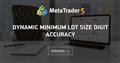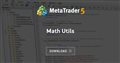# How to get the number of digits of the minimum volume?

Hi,

I'ld like to be able to know the number of decimal digits of the minimum volume/step of a symbol, something like _Digits but for volume. So suppose the min volume is "1": VolDigits = 0. Or min volume is "0.45": VolDigits = 2.

Searching in the forum I did manage to find another thread about this, but not only it's pretty old, but quite confusing. And I couldn't find anything native in MQL5 like _Digits either.

So, how can I do it? I though about transforming the number into a string and working that one, but I'm suspicious that won't work properly.Dynamic minimum lot size digit accuracy
• 2013.02.03
• www.mql5.com
Hello. I'm working to have code to be able to dynamically determine the digit accuracy of the minimum lot size...

Thanks for pointing me that out! Unfortunately there are some many different codes there I really don't know which I should be using, especially given it is in MQL4's forum and not MQL5 ^.^ Do you have a suggestion?

Martin Bittencourt #:

Thanks for pointing me that out! Unfortunately there are some many different codes there I really don't know which I should be using, especially given it is in MQL4's forum and not MQL5 ^.^ Do you have a suggestion?

```int Digits(const double number)
{
//---
double num=number;
int count=0;
for(;count<8;count++) {
if(!((int)num-num))
return(count);
num*=10; }
//---
return(count);
}         ```

Here is another way to ge the digits of a number.

```//+------------------------------------------------------------------+
//| MathDigits                                                       |
//+------------------------------------------------------------------+

// Function
template <typename T>
const int MathDigits(const T value)
{ return((int)MathRound(MathAbs(MathLog10((double)value)))); }

// Inline macro
#define inline_MathDigits(value) ((int)MathRound(MathAbs(MathLog10((double)value))))

```

Please take a look at the Math Utis library

```// Get number of fractional digits after the decimal point.
int    GetDigits(double num);

// Get number of integer digits to the left of decimal point.
int    GetIntegerDigits(double num);
```

```//+------------------------------------------------------------------+
//| Get number of fractional digits after the decimal point.         |
//+------------------------------------------------------------------+
int GetDigits(double num)
{
int d = 0; double p = 1;
while(MathRound(num * p) / p != num)
{
p = MathPow(10, ++d);
}
return d;
}

//+------------------------------------------------------------------+
//|                                                                  |
//+------------------------------------------------------------------+
void OnStart()
{
Print("======== Testing GetDigits() ========");
Print( GetDigits(1.13113) );  // The correct output should be 5
}
```Math Utils
• www.mql5.com
Handy functions for comparison, rounding, formatting and debugging of doubles (prices, lots and money).

Well, thanks people for all your replies! :) I ended up using the following:

```int countDigits(const double val, const int maxPrecision = 8)
{ //https://www.mql5.com/en/forum/385354
int digits = 0;

while (NormalizeDouble(val,digits) != NormalizeDouble(val,maxPrecision))
++digits;

return digits;
}```

Dominik Christian Egert #: Here is another way to ge the digits of a number.

Fails if the number is not an exact power of 10, e.g. 0.5